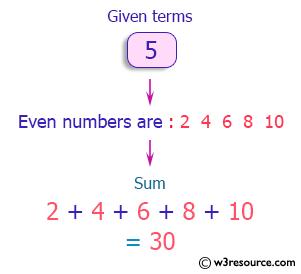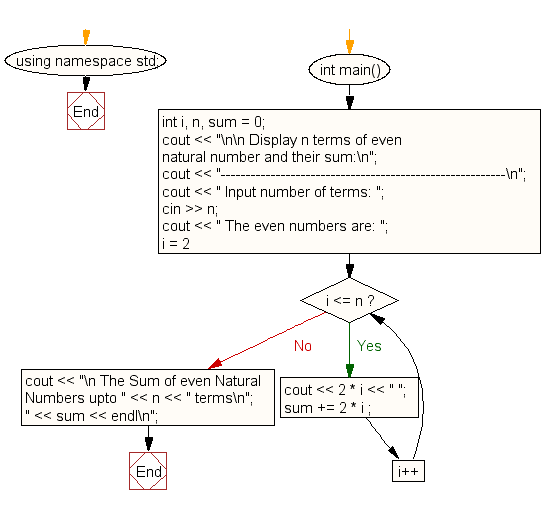﻿ C++ : n terms of even natural number and their sum

# C++ Exercises: Display the n terms of even natural number and their sum

## C++ For Loop: Exercise-21 with Solution

Write a C++ program that displays the sum of the n terms of even natural numbers.

Visual Presentation:Sample Solution:-

C++ Code :

``````#include <iostream> // Including input-output stream header

using namespace std; // Using standard namespace to avoid writing std::

int main() // Start of the main function
{
int i, n, sum = 0; // Declaration of integer variables 'i' (loop counter), 'n' (user input for the number of terms), 'sum' (sum of even natural numbers)

cout << "\n\n Display n terms of even natural number and their sum:\n"; // Display message on the console
cout << "---------------------------------------------------------\n";
cout << " Input number of terms: "; // Prompt the user to input the number of terms
cin >> n; // Read the input value as the number of terms

cout << "\n The even numbers are: "; // Display message indicating the list of even numbers

for (i = 1; i <= n; i++) // Loop for 'n' terms
{
cout << 2 * i << " "; // Print the even numbers by formula 2 * 'i'
sum += 2 * i; // Add the even number to the sum variable
}

cout << "\n The Sum of even Natural Numbers up to " << n << " terms: " << sum << endl; // Display the sum of even numbers up to 'n' terms
}
``````

Sample Output:

```Display n terms of even natural number and their sum:
---------------------------------------------------------
Input number of terms: 5
The even numbers are: 2 4 6 8 10
The Sum of even Natural Numbers upto 5 terms: 30
```

Flowchart:C++ Code Editor: